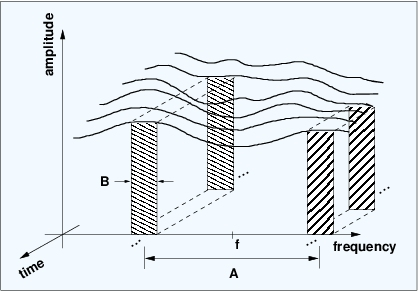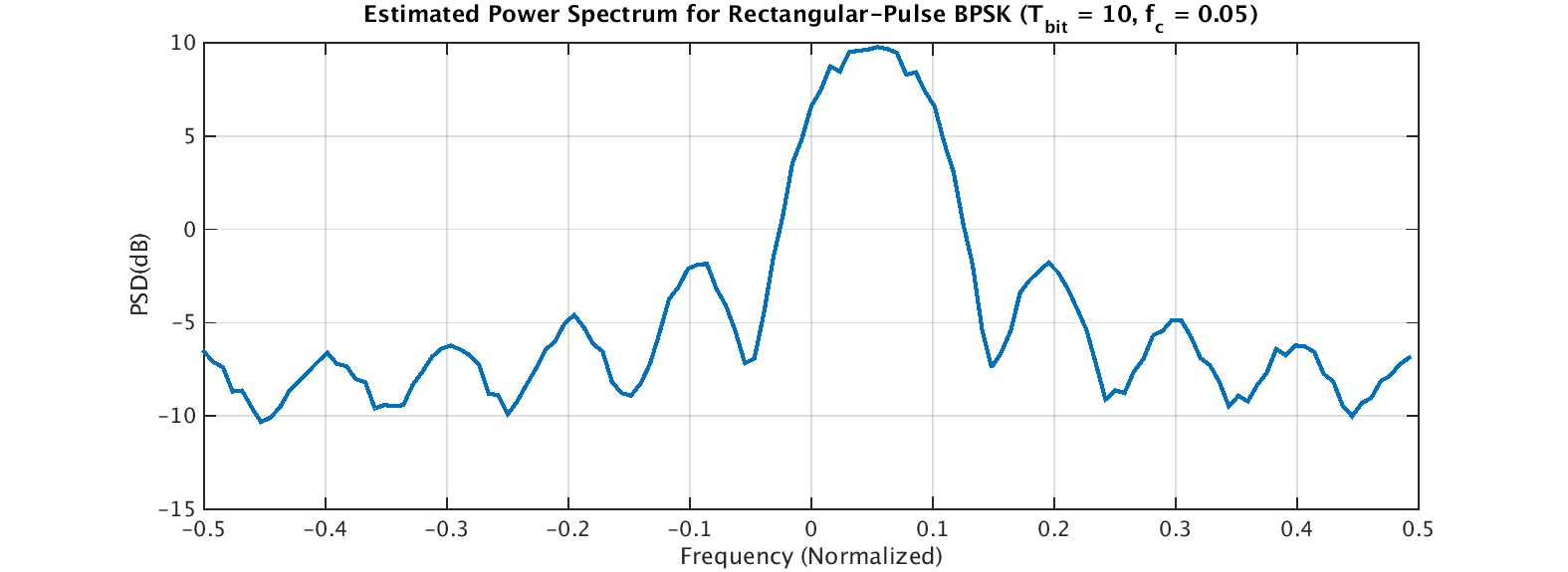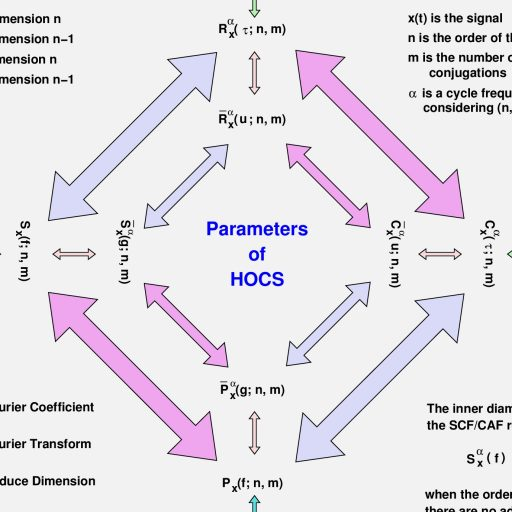## Signal Selectivity

We can estimate the spectral correlation function of one signal in the presence of another with complete temporal and spectral overlap provided the signal has a unique cycle frequency.

In this post I describe and illustrate the most important property of cyclostationary statistics: signal selectivity. The idea is that the cyclostationary parameters for a single signal can be estimated for that signal even when it is corrupted by strong noise and cochannel interferers. ‘Cochannel’ means that the interferer occupies a frequency band that partially or completely overlaps the frequency band for the signal of interest.

A mixture of received RF signals, whether cochannel or not, is accurately modeled by the simple sum of the signals, as in$x(t) = s_1(t) + s_2(t) + \ldots + s_K(t) + w(t), \hfill (1)$

where$w(t)$ is additive noise. We can write this more compactly as$x(t) = \displaystyle \sum_{k=1}^K s_k(t) + w(t). \hfill (2)$

## The Spectral Correlation Function for Rectangular-Pulse BPSK

Let’s make the spectral correlation function a little less abstract by showing it for a simple textbook BPSK signal.

In this post, I show the non-conjugate and conjugate spectral correlation functions (SCFs) for the rectangular-pulse BPSK signal we generated in a previous post. The theoretical SCF can be analytically determined for a rectangular-pulse BPSK signal with independent and identically distributed bits (see My Papers  for example or The Literature [R1]). The cycle frequencies are, of course, equal to those for the CAF for rectangular-pulse BPSK. In particular, for the non-conjugate SCF, we have cycle frequencies of$\alpha = k f_{bit}$ for all integers$k$, and for the conjugate SCF we have$\alpha = 2f_c \pm k f_{bit}$.

## The Spectral Correlation Function

Spectral correlation in CSP means that distinct narrowband spectral components of a signal are correlated-they contain either identical information or some degree of redundant information.

Spectral correlation is perhaps the most widely used characterization of the cyclostationarity property. The main reason is that the computational efficiency of the FFT can be harnessed to characterize the cyclostationarity of a given signal or data set in an efficient manner. And not just efficient, but with a reasonable total computational cost, so that one doesn’t have to wait too long for the result.

Just as the normal power spectrum is actually the power spectral density, or more accurately, the spectral density of time-averaged power (or simply the variance when the mean is zero), the spectral correlation function is the spectral density of time-averaged correlation (covariance). What does this mean? Consider the following schematic showing two narrowband spectral components of an arbitrary signal:Figure 1. Illustration of the concept of spectral correlation. The time series represented by the narrowband spectral components centered at and are downconverted to zero frequency and their correlation is measured. When , the result is the power spectral density function, otherwise it is referred to as the spectral correlation function. It is non-zero only for a countable set of numbers , which are equal to the frequencies of sine waves that can be generated by quadratically transforming the data.

Let’s define narrowband spectral component to mean the output of a bandpass filter applied to a signal, where the bandwidth of the filter is much smaller than the bandwidth of the signal.

The sequence of shaded rectangles on the left are meant to imply a time series corresponding to the output of a bandpass filter centered at$f-A/2$ with bandwidth$B.$ Similarly, the sequence of shaded rectangles on the right imply a time series corresponding to the output of a bandpass filter centered at$f+A/2$ with bandwidth$B.$

## The Cyclic Autocorrelation for Rectangular-Pulse BPSK

Let’s look at a specific example of the cyclic autocorrelation function: the textbook rectangular-pulse BPSK signal with IID symbols.

The cyclic autocorrelation function (CAF) for rectangular-pulse BPSK can be derived as a relatively simple closed-form expression (see My Papers  for example or The Literature [R1]). It can be estimated in a variety of ways, which we will discuss in future posts. The non-conjugate cycle frequencies for the signal are harmonics of the bit rate,$k f_{bit}$, and the conjugate cycle frequencies are the non-conjugate cycle frequencies offset by the doubled carrier, or$2f_c + k f_{bit}$.

Recall that our simulated rectangular-pulse BPSK signal has$10$ samples per bit, or a bit rate of$0.1$, and a carrier offset of$0.05$, all in normalized units (meaning the sampling rate is unity). We’ve previously selected a sampling rate of$1.0$ MHz to provide a little physical realism; let’s do that here too. This choice means the bit rate is$100$ kHz and the carrier offset frequency is$50$ kHz. From these numbers, we see that the non-conjugate cycle frequencies are$k 100$ kHz, and that the conjugate cycle frequencies are$2(50) + k 100$ kHz, or$100 + k 100$ kHz.

## The Cyclic Autocorrelation Function

The cyclic autocorrelation function is the amplitude of a Fourier-series component of the time-varying autocorrelation for a cyclostationary signal.

In this post, I introduce the cyclic autocorrelation function (CAF). The easiest way to do this is to first review the conventional autocorrelation function. Suppose we have a complex-valued signal$x(t)$ defined on a suitable probability space. Then the mean value of$x(t)$ is given by$M_x(t, \tau) = E[x(t + \tau)]. \hfill (1)$

For stationary signals, and many cyclostationary signals, this mean value is independent of the lag parameter$\tau$, so that$\displaystyle M_x(t, \tau_1) = M_x(t, \tau_2) = M_x(t, 0) = M_x. \hfill (2)$

The autocorrelation function is the correlation between the random variables corresponding to two time instants of the random signal, or$\displaystyle R_x(t_1, t_2) = E[x(t_1)x^*(t_2)]. \hfill (3)$

## Creating a Simple CS Signal: Rectangular-Pulse BPSK

We’ll use this simple textbook signal throughout the CSP Blog to illustrate and tie together all the different aspects of CSP.

To test the correctness of various CSP estimators, we need a sampled signal with known cyclostationary parameters. Additionally, the signal should be easy to create and understand. A good candidate for this kind of signal is the binary phase-shift keyed (BPSK) signal with rectangular pulse function.

PSK signals with rectangular pulse functions have infinite bandwidth because the signal bandwidth is determined by the Fourier transform of the pulse, which is a sinc() function for the rectangular pulse. So the rectangular pulse is not terribly practical–infinite bandwidth is bad for other users of the spectrum. However, it is easy to generate, and its statistical properties are known.

So let’s jump in. The baseband BPSK signal is simply a sequence of binary ($\pm$ 1) symbols convolved with the rectangular pulse. The MATLAB script make_rect_bpsk.m does this and produces the following plot:Figure 1. Time-domain plot of a baseband (not yet modulated by a carrier) rectangular-pulse BPSK signal with bit rate 1/10.

The signal alternates between amplitudes of +1 and -1 randomly. After frequency shifting and adding white Gaussian noise, we obtain the power spectrum estimate:Figure 2. Power spectrum estimate for a simulated rectangular-pulse BPSK signal in noise. The signal power is unity, or 0 dB, and the noise power is 1/10, or -10 dB. The bit rate is 1/10 and the carrier offset frequency is 0.05. Note that the nulls (minima) of the signal spectrum are at , or harmonics of the bit rate offset by the carrier.

The power spectrum plot shows why the rectangular-pulse BPSK signal is not popular in practice. The range of frequencies for which the signal possesses non-zero average power is infinite, so it will interfere with signals “nearby” in frequency. However, it is a good signal for us to use as a test input in all of our CSP algorithms and estimators.

The MATLAB script that creates the BPSK signal and the plots above is here. It is an m-file but I’ve stored it in a .doc file due to WordPress limitations I can’t yet get around.

## Welcome to the CSP Blog!Thank you for visiting the CSP blog.

The purpose of this blog is to talk about cyclostationary signals and cyclostationary signal processing (CSP). I’ve been working in the area for nearly thirty years, and over that time I’ve received a lot of requests for help with CSP code and algorithms. I thought it was time to put some of the basics out on the web so everybody could benefit. And I’m hoping to learn from you too.In future posts, I’ll be showing how to create simple cyclostationary signals, write code for basic CSP estimators and detectors, and discuss papers in the literature.

What is cyclostationarity? It is a property of a class of mathematical models for a large number of signals in the world, most notably man-made modulated radio-frequency signals, like those used by cell phones, broadcast AM/FM/TV, satellites, WiFi modems, and many more systems. The mathematical models can be quite accurate, so we also say that cyclostationarity is a property of the real-world signals themselves.

The key aspect of the model is that cyclostationary signals have probabilistic parameters that vary periodically with time. Traditionally, signals are treated as stationary, which means their parameters do not vary with time. What are these ‘probabilistic parameters?’ Quantities like the mean value, the variance, and higher-order moments. These quantities are defined for both the time-domain signal and for its frequency-domain representation. So we have ‘temporal moments‘ and ‘spectral moments.’ The second-order spectral moment is also called the spectral correlation function (SCF). The SCF is central to many CSP algorithms; a display of the SCF is shown above for a simple bandlimited binary phase-shift keyed (BPSK) signal.

The well-known noise- and interference-tolerance properties of CSP algorithms follow from the periodically time-varying nature of the signals’ parameters.

The most common difficulty I’ve encountered is when a researcher is developing a CSP estimator and is having trouble applying it to their data set. The researchers almost always skip the step of first applying the estimator to a signal with a perfectly known cyclostationary parameters. So, in the next post I’ll describe how to make the simplest digital CS signal, which has known temporal and spectral moments of all orders, so that we can test CSP estimators by comparing their output to the known correct result.

I encourage readers to point out my errors in the comments of my posts and to suggest topics they would like to see covered in future posts. Also, let me know about your application and interests so I can continue to learn too.

I hope you enjoy your time here at the CSP Blog!Support the CSP Blog and Keep it Ad-Free

Please consider donating to the CSP Blog to keep it ad-free and to support the addition of major new features. The small box below is used to specify the number of $5 donations.$5.00